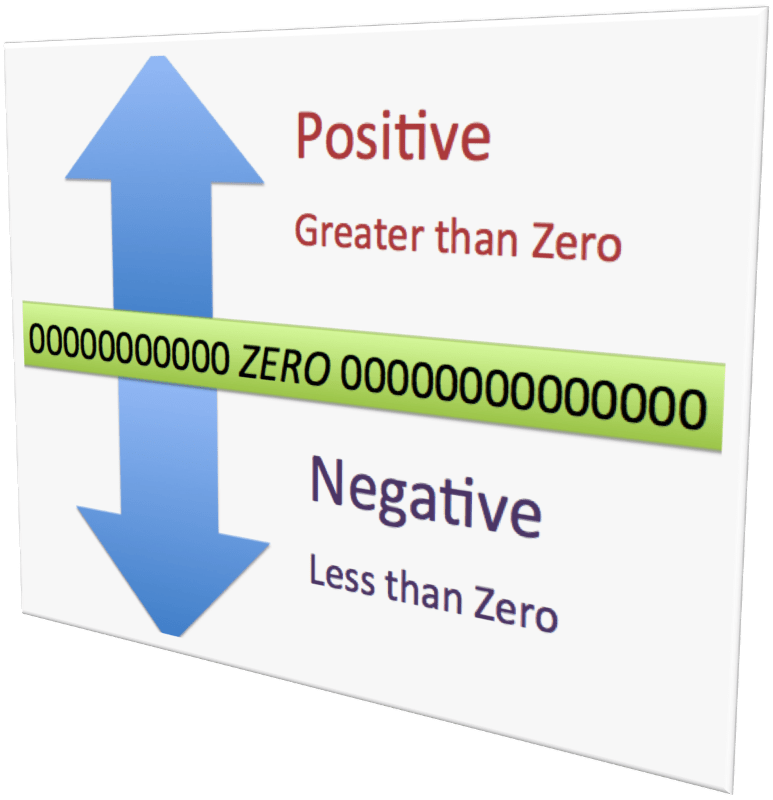# Representing Numbers on a Number LinePositive numbers are greater than 0.   They may be written with a positive sign, but are usually written without it.   For example, a gain of 5 yards in football can be written as +5 or 5.

Negative numbers are less than 0.   They are always written with a negative sign (-).   For example, a temperature of 10 degrees below zero is written as -10o.

You can graph positive and negative numbers on a number line.   The positive numbers are to the right of zero and the negative numbers are to the left of 0.

A number line can also be used to compare and order numbers.   On a number line, the number to the left is always less than the number to the right.You can have fractions and decimals on a number and as negatives just like integers.## Practice

Representing Numbers on a Number Line Assignment
1. Write a number to describe gaining 4 pounds.
2. Write a number to describe a \$15 withdrawal.
3. Write a number to describe a \$40 deposit into an account.
Replace the blank with < or > to make a true sentence.
4. -3 ___ -5
5. 3 ___ 5
6. 0 ___ -4
7. -2 ___ 3
Order the numbers from least to greatest.
8. -8, 3, -4, and 5
9. -1, 6, 0, and -3
10. -6 ½, 2, 4.5, and -4

(source)